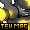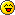Where you can chat, trade, battle, and tons more!Rukario Forums :: Introduce Yourself! :: Leaving? Share

# pfffffffffffffffffffffffffffffffffffffffffffffffffffffffffffffffftAuthorMessagePosts : 56
Join date : 2009-08-11
Age : 22Subject: pfffffffffffffffffffffffffffffffffffffffffffffffffffffffffffffffftMon Aug 17, 2009 9:18 am no body post here.im already sick of this place, all the nubs, spam, useless post's.kthxbaiPosts : 134
Join date : 2009-08-11
Age : 26
Location : Some where in Texas.Subject: Re: pfffffffffffffffffffffffffffffffffffffffffffffffffffffffffffffffftMon Aug 17, 2009 9:04 pm Teh~Mag wrote:no body post here.im already sick of this place, all the nubs, spam, useless post's.kthxbai Bye ***holePosts : 56
Join date : 2009-08-11
Age : 22Subject: Re: pfffffffffffffffffffffffffffffffffffffffffffffffffffffffffffffffftTue Aug 18, 2009 12:07 pm You just proved my point of spam and useless post's there, nice one idiot.Posts : 39
Join date : 2009-08-17
Age : 25
Location : My room :PSubject: Re: pfffffffffffffffffffffffffffffffffffffffffffffffffffffffffffffffftTue Aug 18, 2009 2:56 pm Okay have a nice Life! CyaPosts : 56
Join date : 2009-08-11
Age : 22Subject: Re: pfffffffffffffffffffffffffffffffffffffffffffffffffffffffffffffffftTue Aug 18, 2009 4:43 pm I SAID DON'T POSTPosts : 39
Join date : 2009-08-17
Age : 25
Location : My room :PSubject: Re: pfffffffffffffffffffffffffffffffffffffffffffffffffffffffffffffffftThu Aug 20, 2009 12:57 am Teh~Mag wrote:I SAID DON'T POSTYou also said you were leaving...Sponsored contentSubject: Re: pfffffffffffffffffffffffffffffffffffffffffffffffffffffffffffffffftpfffffffffffffffffffffffffffffffffffffffffffffffffffffffffffffffftPage 1 of 1

Permissions in this forum:You cannot reply to topics in this forumJump to: Select a forum||--Introduce Yourself!|   |--Introductions|   |--Leaving?|   |--Events|   |--Announcements|   |--Nintendo DS Games|   |--Pokemon Games|   |--Naruto Games|   |--Yugioh Games|   |--DS Wi-Fi|   |--Other Games|   |--Nintendo Wii Games|   |--Mario Kart Wii|   |--Pokemon Games|   |--Super Smash Bros. Brawl|   |--Naruto|   |--Wii Wi-Fi|   |--Other Games|   |--Youtube|   |--General Youtube Discussion|   |--Suggestions    |--Forum Suggestions    |--Youtube Suggestions